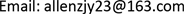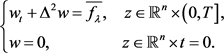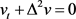﻿ 双调和抛物方程解的局部Lorentz估计 Local Lorentz Estimates for the Bi-Harmonic Parabolic Equation

Pure Mathematics
Vol. 09  No. 05 ( 2019 ), Article ID: 31402 , 10 pages
10.12677/PM.2019.95080

Local Lorentz Estimates for the Bi-Harmonic Parabolic Equation

Jiayi Zhu

College of Sciences, Shanghai University, ShanghaiReceived: Jun. 30th, 2019; accepted: Jul. 18th, 2019; published: Jul. 25th, 2019ABSTRACT

Lp estimates are the basic regularity estimates in the partial differential equations. In this paper, we mainly study a new class of regularity estimates—Lorentz estimates for the Bi-harmonic parabolic equation.

Keywords:Bi-Harmonic Parabolic Equation, Regularity Estimates, Lorentz SpaceLp估计是偏微分方程中基本的正则性估计，本文我们主要研究一类四阶双调和抛物方程的解的新的正则性估计——Lorentz估计。1. 引言

${u}_{t}+{\Delta }^{2}u=f,\text{\hspace{0.17em}}z\in {Q}_{2}\subset {ℝ}^{n+1}$ (1)

$|{D}^{4}u|=|{D}_{x}^{4}u\left(x,t\right)|={\left(\underset{i,j,k,l=1,\cdots ,n}{\sum }{|{D}_{{x}_{i}{x}_{j}{x}_{k}{x}_{l}}u\left(x,t\right)|}^{2}\right)}^{\frac{1}{2}},$

${‖{D}^{4}u‖}_{{L}^{p}\left({ℝ}^{n+1}\right)}=\underset{i,j,k,l=1,\cdots ,n}{\sum }{‖{D}_{{x}_{i}{x}_{j}{x}_{k}{x}_{l}}u\left(x,t\right)‖}_{{L}^{p}\left({ℝ}^{n+1}\right)}.$

${‖{D}^{4}u‖}_{L\left(\gamma ,q\right)\left({Q}_{1}\right)}\le c\left({‖{D}^{4}u‖}_{{L}^{2}\left({Q}_{2}\right)}+{‖f‖}_{L\left(\gamma ,q\right)\left({Q}_{2}\right)}\right).$

${‖g‖}_{L\left(\gamma ,q\right)\left(Q\right)}^{q}:=q{\int }_{0}^{\infty }{\lambda }^{q-1}{|\left\{\xi \in Q:|g\left(\xi \right)|>\lambda \right\}|}^{\frac{q}{\gamma }}\text{d}\lambda <+\infty .$

${‖g‖}_{L\left(\gamma ,\infty \right)\left(Q\right)}={‖g‖}_{{\mathcal{M}}^{\gamma }\left(Q\right)}:=\underset{\lambda >0}{\mathrm{sup}}{\left({\lambda }^{\gamma }|\left\{\xi \in Q:|g\left(\xi \right)|>\lambda \right\}|\right)}^{\frac{1}{\gamma }}<+\infty .$

 (2)

 (3)

${‖{D}^{4}u‖}_{L\left(\gamma ,q\right)\left({Q}_{1}\right)}\le c\left({‖{D}^{4}u‖}_{{L}^{2}\left({Q}_{2}\right)}+{‖f‖}_{L\left(\gamma ,q\right)\left({Q}_{2}\right)}\right),$ (4)

2. 引理和准备工作

${\int }_{0}^{\infty }F\left(\lambda \right)\text{d}\lambda <\infty .$

${\int }_{0}^{\infty }{\lambda }^{r}{\left({\int }_{\lambda }^{\infty }F\left(\mu \right)\text{d}\mu \right)}^{\alpha }\frac{\text{d}\lambda }{\lambda }\le {\left(\frac{\alpha }{r}\right)}^{\alpha }{\int }_{0}^{\infty }{\lambda }^{r}{\left[\lambda F\left(\lambda \right)\right]}^{\alpha }\frac{\text{d}\lambda }{\lambda }.$

${\left[{\int }_{\lambda }^{\infty }{\left[{\mu }^{r}h\left(\mu \right)\right]}^{{\alpha }_{2}}\frac{\text{d}\mu }{\mu }\right]}^{\frac{1}{{\alpha }_{2}}}\le \epsilon {\lambda }^{r}h\left(\lambda \right)+\frac{c}{{\epsilon }^{\frac{{\alpha }_{2}}{{\alpha }_{1}}-1}}{\left[{\int }_{\lambda }^{\infty }{\left[{\mu }^{r}h\left(\mu \right)\right]}^{{\alpha }_{1}}\frac{\text{d}\mu }{\mu }\right]}^{\frac{1}{{\alpha }_{1}}},$

$\underset{\mu >\lambda }{\mathrm{sup}}\left[{\mu }^{r}h\left(\mu \right)\right]\le c{\lambda }^{r}h\left(\lambda \right)+c{\left({\int }_{\lambda }^{\infty }{\left[{\mu }^{r}h\left(\mu \right)\right]}^{{\alpha }_{1}}\frac{\text{d}\mu }{\mu }\right)}^{\frac{1}{{\alpha }_{1}}},$

$\phi \left(t\right)\le \theta \phi \left(s\right)+\frac{A}{{\left(s-t\right)}^{\alpha }}+B,$

$\phi \left(\rho \right)\le c\left(\frac{A}{{\left(R-\rho \right)}^{\alpha }}+B\right),$

${‖f‖}_{{L}^{q}\left(Q\right)}\le {\left(\frac{t}{t-q}\right)}^{\frac{1}{q}}{|Q|}^{\frac{1}{q}-\frac{1}{t}}{‖f‖}_{{\mathcal{M}}^{t}\left(Q\right)}.$

${\lambda }_{0}={\left(\frac{1}{|{Q}_{2}|}{\int }_{{Q}_{2}}{|{D}^{4}u|}^{2}\text{d}z\right)}^{\frac{1}{2}}+{\left({M}^{\frac{\eta }{2}}\frac{1}{|{Q}_{2}|}{\int }_{{Q}_{2}}{|f|}^{\eta }\text{d}z\right)}^{\frac{1}{\eta }}.$

$CZ\left({Q}_{r}\left({z}_{i}\right)\right):={\left(\frac{1}{|{Q}_{r}\left({z}_{i}\right)|}{\int }_{{Q}_{r}\left({z}_{i}\right)}{|{D}^{4}u|}^{2}\text{d}z\right)}^{\frac{1}{2}}+{\left({M}^{\frac{\eta }{2}}\frac{1}{|{Q}_{r}\left({z}_{i}\right)|}{\int }_{{Q}_{r}\left({z}_{i}\right)}{|f|}^{\eta }\text{d}z\right)}^{\frac{1}{\eta }},$

$\begin{array}{c}CZ\left({Q}_{r}\left({z}_{i}\right)\right)={\left(\frac{1}{|{Q}_{r}\left({z}_{i}\right)|}{\int }_{{Q}_{r}\left({z}_{i}\right)}{|{D}^{4}u|}^{2}\text{d}z\right)}^{\frac{1}{2}}+{\left({M}^{\frac{\eta }{2}}\frac{1}{|{Q}_{r}\left({z}_{i}\right)|}{\int }_{{Q}_{r}\left({z}_{i}\right)}{|f|}^{\eta }\text{d}z\right)}^{\frac{1}{\eta }}\\ \le {\left(\frac{|{Q}_{2}|}{|{Q}_{r}\left({z}_{i}\right)|}\right)}^{\frac{1}{2}}\left[{\left(\frac{1}{|{Q}_{2}|}{\int }_{{Q}_{2}}{|{D}^{4}u|}^{2}\text{d}z\right)}^{\frac{1}{2}}+{\left({M}^{\frac{\eta }{2}}\frac{1}{|{Q}_{2}|}{\int }_{{Q}_{2}}{|f|}^{\eta }\text{d}z\right)}^{\frac{1}{\eta }}\right]\\ \le {40}^{\frac{4+n}{2}}{\lambda }_{0}<\lambda .\end{array}$

$CZ\left({Q}_{{r}_{i}}\left({z}_{i}\right)\right)={\left(\frac{1}{|{Q}_{{r}_{i}}\left({z}_{i}\right)|}{\int }_{{Q}_{{r}_{i}}\left({z}_{i}\right)}{|{D}^{4}u|}^{2}\text{d}z\right)}^{\frac{1}{2}}+{\left({M}^{\frac{\eta }{2}}\frac{1}{|{Q}_{{r}_{i}}\left({z}_{i}\right)|}{\int }_{{Q}_{{r}_{i}}\left({z}_{i}\right)}{|f|}^{\eta }\text{d}z\right)}^{\frac{1}{\eta }}=\lambda ,$ (5)

$CZ\left({Q}_{r}\left({z}_{i}\right)\right)={\left(\frac{1}{|{Q}_{r}\left({z}_{i}\right)|}{\int }_{{Q}_{r}\left({z}_{i}\right)}{|{D}^{4}u|}^{2}\text{d}z\right)}^{\frac{1}{2}}+{\left({M}^{\frac{\eta }{2}}\frac{1}{|{Q}_{r}\left({z}_{i}\right)|}{\int }_{{Q}_{r}\left({z}_{i}\right)}{|f|}^{\eta }\text{d}z\right)}^{\frac{1}{\eta }}<\lambda .$ (6)

${\left(\frac{\lambda }{2}\right)}^{2}\le \frac{1}{|Q|}{\int }_{Q}{|{D}^{4}u|}^{2}\text{d}z$${\left(\frac{\lambda }{2}\right)}^{\eta }\le {M}^{\frac{\eta }{2}}\frac{1}{|Q|}{\int }_{Q}{|f|}^{\eta }\text{d}z.$ (7)

$\begin{array}{c}{\left(\frac{\lambda }{2}\right)}^{2}\le \frac{1}{|Q|}{\int }_{Q}{|{D}^{4}u|}^{2}\text{d}z=2{\int }_{0}^{\infty }\mu |\left\{z\in Q:|{D}^{4}u|>\mu \right\}|\text{d}\mu \\ \le 2{\int }_{0}^{\frac{\lambda }{4}}\mu |\left\{z\in Q:|{D}^{4}u|>\mu \right\}|\text{d}\mu +2{\int }_{\frac{\lambda }{4}}^{\infty }\mu |\left\{z\in Q:|{D}^{4}u|>\mu \right\}|\text{d}\mu \\ \le \frac{{\lambda }^{2}}{16}|Q|+2{\int }_{\frac{\lambda }{4}}^{\infty }\mu |\left\{z\in Q:|{D}^{4}u|>\mu \right\}|\text{d}\mu \end{array}$

$|Q|\le \frac{c}{{\lambda }^{2}}{\int }_{\frac{\lambda }{4}}^{\infty }\mu |\left\{z\in Q:|{D}^{4}u|>\mu \right\}|\text{d}\mu .$ (8)

$\begin{array}{c}{\left(\frac{\lambda }{2}\right)}^{\eta }\frac{1}{{M}^{\eta /2}}\le \frac{1}{|Q|}{\int }_{Q}{|f|}^{\eta }\text{d}z=\frac{\eta }{|Q|}{\int }_{0}^{\infty }{\mu }^{\eta -1}|\left\{z\in Q:|f|>\mu \right\}|\text{d}\mu \\ \le {\left(\zeta \lambda \right)}^{\eta }+\frac{\eta }{|Q|}{\int }_{\zeta \lambda }^{\infty }{\mu }^{\eta -1}|\left\{z\in Q:|f|>\mu \right\}|\text{d}\mu \end{array}$

$|Q|\le \frac{c}{{\lambda }^{\eta }}{\int }_{\zeta \lambda }^{\infty }{\mu }^{\eta -1}|\left\{z\in Q:|f|>\mu \right\}|\text{d}\mu .$ (9)

$|Q|\le \frac{c}{{\lambda }^{2}}{\int }_{\frac{\lambda }{4}}^{\infty }\mu |\left\{z\in Q:|{D}^{4}u|>\mu \right\}|\text{d}\mu +\frac{c}{{\lambda }^{\eta }}{\int }_{\zeta \lambda }^{\infty }{\mu }^{\eta -1}|\left\{z\in Q:|f|>\mu \right\}|\text{d}\mu .$ (10)

${u}_{\lambda }=u/\lambda$${f}_{\lambda }=f/\lambda$ 。事实上，根据式(6)可得如下两个不等式

$\frac{1}{|{Q}_{10{r}_{i}}\left({z}_{i}\right)|}{\int }_{{Q}_{10{r}_{i}}\left({z}_{i}\right)}{|{D}^{4}{u}_{\lambda }|}^{2}\text{d}z\le 1,$ (11)

$\frac{1}{|{Q}_{10{r}_{i}}\left({z}_{i}\right)|}{\int }_{{Q}_{10{r}_{i}}\left({z}_{i}\right)}{|{f}_{\lambda }|}^{2}\text{d}z\le c{\left(\frac{1}{|{Q}_{10{r}_{i}}\left({z}_{i}\right)|}{\int }_{{Q}_{10{r}_{i}}\left({z}_{i}\right)}{|{f}_{\lambda }|}^{\eta }\text{d}z\right)}^{\frac{2}{\eta }}\le \frac{c}{M}.$ (12)${L}^{p}$ 估计  ，我么可得： ${‖{D}^{4}w‖}_{{L}^{2}\left({ℝ}^{n}×\left(0,T\right]\right)}\le c{‖\stackrel{¯}{{f}_{\lambda }}‖}_{{L}^{2}\left({ℝ}^{n}×\left(0,T\right]\right)}$ 。从而可得

${\int }_{{Q}_{10{r}_{i}}\left({z}_{i}\right)}{|{D}^{4}w|}^{2}\text{d}z\le {\int }_{{ℝ}^{n}×\left(0,T\right]}{|{D}^{4}w|}^{2}\text{d}z\le c{\int }_{{ℝ}^{n}×\left(0,T\right]}{|\stackrel{¯}{{f}_{\lambda }}|}^{2}\text{d}z=c{\int }_{{Q}_{10{r}_{i}}\left({z}_{i}\right)}{|\stackrel{¯}{{f}_{\lambda }}|}^{2}\text{d}z,$

$\begin{array}{l}\frac{1}{|{Q}_{10{r}_{i}}\left({z}_{i}\right)|}{\int }_{{Q}_{10{r}_{i}}\left({z}_{i}\right)}{|{D}^{4}w|}^{2}\text{d}z\\ \le c\frac{1}{|{Q}_{10{r}_{i}}\left({z}_{i}\right)|}{\int }_{{Q}_{10{r}_{i}}\left({z}_{i}\right)}{|{f}_{\lambda }|}^{2}\text{d}z\\ \le c{\left(\frac{1}{|{Q}_{10{r}_{i}}\left({z}_{i}\right)|}{\int }_{{Q}_{10{r}_{i}}\left({z}_{i}\right)}{|{f}_{\lambda }|}^{\eta }\text{d}z\right)}^{\frac{2}{\eta }}\le \frac{c}{M}\le c\end{array}$ (13)

$v={u}_{\lambda }-w$ ，可知$z\in {Q}_{10{r}_{i}}\left({z}_{i}\right)$

$\begin{array}{l}\frac{1}{|{Q}_{10{r}_{i}}\left({z}_{i}\right)|}{\int }_{{Q}_{10{r}_{i}}\left({z}_{i}\right)}{|{D}^{4}v|}^{2}\text{d}z\\ \le 2\left[\frac{1}{|{Q}_{10{r}_{i}}\left({z}_{i}\right)|}{\int }_{{Q}_{10{r}_{i}}\left({z}_{i}\right)}{|{D}^{4}w|}^{2}\text{d}z+\frac{1}{|{Q}_{10{r}_{i}}\left({z}_{i}\right)|}{\int }_{{Q}_{10{r}_{i}}\left({z}_{i}\right)}{|{D}^{4}{u}_{\lambda }|}^{2}\text{d}z\right]\le c\end{array}$

${z}_{i}\in {Q}_{5{r}_{i}}\left({z}_{i}\right)\cap E\left(A\lambda ,{Q}_{1}\right)$ ，结合式(12)得到

$\begin{array}{l}|\left\{z\in {Q}_{5{r}_{i}}\left({z}_{i}\right):|{D}^{4}u|>A\lambda \right\}|=|\left\{z\in {Q}_{5{r}_{i}}\left({z}_{i}\right):|{D}^{4}{u}_{\lambda }|>A\right\}|\\ \le |\left\{z\in {Q}_{5{r}_{i}}\left({z}_{i}\right):|{D}^{4}w|>\frac{A}{2}\right\}|+|\left\{z\in {Q}_{5{r}_{i}}\left({z}_{i}\right):|{D}^{4}v|>\frac{A}{2}\right\}|\\ =|\left\{z\in {Q}_{5{r}_{i}}\left({z}_{i}\right):|{D}^{4}w|>\frac{A}{2}\right\}|\le \frac{c}{{A}^{2}}{\int }_{{Q}_{5{r}_{i}}\left({z}_{i}\right)}{|{D}^{4}w|}^{2}\text{d}z\\ \le {\int }_{{Q}_{10{r}_{i}}\left({z}_{i}\right)}{|{f}_{\lambda }|}^{2}\text{d}z\le \frac{c}{M}|{Q}_{10{r}_{i}}\left({z}_{i}\right)|\le \frac{c}{M}|{Q}_{10{r}_{i}}\left({z}_{i}\right)|\end{array}$ (14)

$E\left(A\lambda ,{Q}_{1}\right)\subset \underset{i\in ℕ}{\cup }{Q}_{5{r}_{i}}\left({z}_{i}\right)\cup \left\{零测集\right\}$ 。再由式(10)和(14)得到

$\begin{array}{l}|\left\{z\in {Q}_{1}:|{D}^{4}u|>A\lambda \right\}|\\ \le \underset{i=1}{\overset{\infty }{\sum }}|\left\{z\in {Q}_{5{r}_{i}}\left({z}_{i}\right):|{D}^{4}u|>A\lambda \right\}|\le \underset{i=1}{\overset{\infty }{\sum }}\frac{c}{M}|{Q}_{{r}_{i}}\left({z}_{i}\right)|\\ \le \frac{c}{M}\underset{i=1}{\overset{\infty }{\sum }}\left[\frac{1}{{\lambda }^{2}}{\int }_{\frac{\lambda }{4}}^{\infty }\mu |\left\{z\in {Q}_{{r}_{i}}\left({z}_{i}\right):|{D}^{4}u|>\mu \right\}|\text{d}\mu +\frac{1}{{\lambda }^{\eta }}{\int }_{\zeta \lambda }^{\infty }{\mu }^{\eta -1}|\left\{z\in {Q}_{{r}_{i}}\left({z}_{i}\right):|f|>\mu \right\}|\text{d}\mu \right]\\ \le \frac{c}{M}\left[\frac{1}{{\lambda }^{2}}{\int }_{\frac{\lambda }{4}}^{\infty }\mu |\left\{z\in {Q}_{2}:|{D}^{4}u|>\mu \right\}|\text{d}\mu +\frac{1}{{\lambda }^{\eta }}{\int }_{\zeta \lambda }^{\infty }{\mu }^{\eta -1}|\left\{z\in {Q}_{2}:|f|>\mu \right\}|\text{d}\mu \right]\end{array}$ . (15)

3. 定理3的证明

$\begin{array}{c}{‖{D}^{4}u‖}_{L\left(\gamma ,q\right)\left({Q}_{1}\right)}^{q}=q{A}^{q}{\int }_{0}^{\infty }{\lambda }^{q-1}{|\left\{z\in {Q}_{1}:|{D}^{4}u|>A\lambda \right\}|}^{\frac{q}{\gamma }}\text{d}\lambda \\ =q{A}^{q}{\int }_{0}^{B{\lambda }_{0}}{\lambda }^{q-1}{|\left\{z\in {Q}_{1}:|{D}^{4}u|>A\lambda \right\}|}^{\frac{q}{\gamma }}\text{d}\lambda \\ \text{\hspace{0.17em}}\text{\hspace{0.17em}}\text{ }+q{A}^{q}{\int }_{B{\lambda }_{0}}^{\infty }{\lambda }^{q-1}{|\left\{z\in {Q}_{1}:|{D}^{4}u|>A\lambda \right\}|}^{\frac{q}{\gamma }}\text{d}\lambda \\ :={S}_{1}+{S}_{2}\end{array}$

$\begin{array}{c}{S}_{1}=q{A}^{q}{\int }_{0}^{B{\lambda }_{0}}{\lambda }^{q-1}{|\left\{z\in {Q}_{1}:|{D}^{4}u|>A\lambda \right\}|}^{\frac{q}{\gamma }}\text{d}\lambda \\ \le c{\left[B{\lambda }_{0}\right]}^{q}\le c{\left[{\left(\frac{1}{|{Q}_{2}|}{\int }_{{Q}_{2}}{|{D}^{4}u|}^{2}\text{d}z\right)}^{\frac{1}{2}}+{M}^{\frac{1}{2}}{\left(\frac{1}{|{Q}_{2}|}{\int }_{{Q}_{2}}{|f|}^{\eta }\text{d}z\right)}^{\frac{1}{\eta }}\right]}^{q}\\ \le c\left[{‖{D}^{4}u‖}_{{L}^{2}\left({Q}_{2}\right)}^{q}+{‖f‖}_{{\mathcal{M}}^{\gamma }\left({Q}_{2}\right)}^{q}\right]\\ \le c\left[{‖{D}^{4}u‖}_{{L}^{2}\left({Q}_{2}\right)}^{q}+{‖f‖}_{L\left(\gamma ,q\right)\left({Q}_{2}\right)}^{q}\right]\end{array}$ (16)

$\begin{array}{c}{S}_{2}\le c{\left(\frac{1}{M}\right)}^{\frac{q}{\gamma }}\left[{\int }_{B{\lambda }_{0}}^{\infty }{\lambda }^{q-1-\frac{2q}{\gamma }}{\left({\int }_{\frac{\lambda }{4}}^{\infty }\mu |\left\{z\in {Q}_{2}:|{D}^{4}u|>\mu \right\}|\text{d}\mu \right)}^{\frac{q}{\gamma }}\text{d}\lambda \\ \text{\hspace{0.17em}}\text{\hspace{0.17em}}+{\int }_{B{\lambda }_{0}}^{\infty }{\lambda }^{q-1-\frac{\eta q}{\gamma }}{\left({\int }_{\zeta \lambda }^{\infty }{\mu }^{\eta -1}|\left\{z\in {Q}_{2}:|f|>\mu \right\}|\text{d}\mu \right)}^{\frac{q}{\gamma }}\text{d}\lambda \right]\end{array}$ (17)

$\begin{array}{c}{S}_{2}\le c{\left(\frac{1}{M}\right)}^{\frac{q}{\gamma }}\left[{\int }_{0}^{\infty }{\lambda }^{q-1-\frac{2q}{\gamma }}{\lambda }^{\frac{q}{\gamma }}{\left(\lambda |\left\{z\in {Q}_{2}:|{D}^{4}u|>\lambda \right\}|\right)}^{\frac{q}{\gamma }}\text{d}\lambda +{\int }_{0}^{\infty }{\lambda }^{q-1-\frac{\eta q}{\gamma }}{\lambda }^{\frac{q}{\gamma }}{\left({\lambda }^{\eta -1}|\left\{z\in {Q}_{2}:|f|>\lambda \right\}|\right)}^{\frac{q}{\gamma }}\text{d}\lambda \right]\\ \le c{\left(\frac{1}{M}\right)}^{\frac{q}{\gamma }}\left[{‖{D}^{4}u‖}_{L\left(\gamma ,q\right)\left({Q}_{2}\right)}^{q}+{‖f‖}_{L\left(\gamma ,q\right)\left({Q}_{2}\right)}^{q}\right]\end{array}$ (18)

$0 ，利用引理5 $\left({\alpha }_{1}=1,{\alpha }_{2}=\frac{\gamma }{q},r=\frac{2q}{\gamma },\epsilon =1\right)$ 得到

$\begin{array}{l}{\left({\int }_{\frac{\lambda }{4}}^{\infty }\mu |\left\{z\in {Q}_{2}:|{D}^{4}u|>\mu \right\}|\text{d}\mu \right)}^{\frac{q}{\gamma }}\\ \le c{\lambda }^{\frac{2q}{\gamma }}{|\left\{z\in {Q}_{2}:|{D}^{4}u|>\frac{\lambda }{4}\right\}|}^{\frac{q}{\gamma }}+c{\int }_{\frac{\lambda }{4}}^{\infty }{\mu }^{\frac{2q}{\gamma }-1}{|\left\{z\in {Q}_{2}:|{D}^{4}u|>\mu \right\}|}^{\frac{q}{\gamma }}\text{d}\mu \end{array}$

$\begin{array}{l}{\int }_{B{\lambda }_{0}}^{\infty }{\lambda }^{q-1-\frac{2q}{\gamma }}{\left({\int }_{\frac{\lambda }{4}}^{\infty }\mu |\left\{z\in {Q}_{2}:|{D}^{4}u|>\mu \right\}|\text{d}\mu \right)}^{\frac{q}{\gamma }}\text{d}\lambda \\ \le c{\int }_{0}^{\infty }{\lambda }^{q-1}{|\left\{z\in {Q}_{2}:|{D}^{4}u|>\frac{\lambda }{4}\right\}|}^{\frac{q}{\gamma }}\text{d}\lambda +c{\int }_{0}^{\infty }{\lambda }^{q-1-\frac{2q}{\gamma }}{\int }_{\frac{\lambda }{4}}^{\infty }{\mu }^{\frac{2q}{\gamma }-1}{|\left\{z\in {Q}_{2}:|{D}^{4}u|>\mu \right\}|}^{\frac{q}{\gamma }}\text{d}\mu \text{d}\lambda \\ \le c{‖{D}^{4}u‖}_{L\left(\gamma ,q\right)\left({Q}_{2}\right)}^{q}+c{\int }_{0}^{\infty }{\mu }^{\frac{2q}{\gamma }-1}{|\left\{z\in {Q}_{2}:|{D}^{4}u|>\mu \right\}|}^{\frac{q}{\gamma }}{\int }_{0}^{4\mu }{\lambda }^{q-1-\frac{2q}{\gamma }}\text{d}\lambda \text{d}\mu \\ \le c{‖{D}^{4}u‖}_{L\left(\gamma ,q\right)\left({Q}_{2}\right)}^{q}\end{array}$ (19)

${\int }_{B{\lambda }_{0}}^{\infty }{\lambda }^{q-1-\frac{\eta q}{\gamma }}{\left({\int }_{\zeta \lambda }^{\infty }{\mu }^{\eta -1}|\left\{z\in {Q}_{2}:|f|>\mu \right\}|\text{d}\mu \right)}^{\frac{q}{\gamma }}\text{d}\lambda \le c{‖f‖}_{L\left(\gamma ,q\right)\left({Q}_{2}\right)}^{q}.$ (20)

${S}_{2}\le c{\left(\frac{1}{M}\right)}^{\frac{q}{\gamma }}\left[{‖{D}^{4}u‖}_{L\left(\gamma ,q\right)\left({Q}_{2}\right)}^{q}+{‖f‖}_{L\left(\gamma ,q\right)\left({Q}_{2}\right)}^{q}\right].$

${‖{D}^{4}u‖}_{L\left(\gamma ,q\right)\left({Q}_{1}\right)}^{q}\le c{\left(\frac{1}{M}\right)}^{\frac{q}{\gamma }}{‖{D}^{4}u‖}_{L\left(\gamma ,q\right)\left({Q}_{2}\right)}^{q}+c{‖{D}^{4}u‖}_{{L}^{2}\left({Q}_{2}\right)}^{q}+c{‖f‖}_{L\left(\gamma ,q\right)\left({Q}_{2}\right)}^{q}.$

${\left(\frac{\lambda }{2}\right)}^{\eta }\le \frac{{M}^{\eta /2}}{|Q|}{\int }_{Q}{|f|}^{\eta }\text{d}z\le {M}^{\eta /2}{\left(\tau \lambda \right)}^{\eta }+\frac{{M}^{\eta /2}}{|Q|}\underset{\left\{z\in Q:|f|>\tau \lambda \right\}}{\int }{|f|}^{\eta }\text{d}z$

$\begin{array}{l}{\left(\frac{\lambda }{2}\right)}^{\eta }-{M}^{\eta /2}{\left(\tau \lambda \right)}^{\eta }\le \frac{{M}^{\eta /2}}{|Q|}\underset{{F}_{1}\left(\tau \lambda ,Q\right)}{\int }{|f|}^{\eta }\text{d}z\\ \le c{M}^{\eta /2}\frac{{|{F}_{1}\left(\tau \lambda ,Q\right)|}^{1-\frac{\eta }{\gamma }}}{|Q|}\underset{\mu >0}{\mathrm{sup}}{\mu }^{\eta }{|\left\{z\in {F}_{1}\left(\tau \lambda ,Q\right):|f|>\mu \right\}|}^{\frac{\eta }{\gamma }}\\ \le c{M}^{\eta /2}\frac{{|{F}_{1}\left(\tau \lambda ,Q\right)|}^{1-\frac{\eta }{\gamma }}}{|Q|}\left[{\left(\tau \lambda \right)}^{\eta }{|{F}_{1}\left(\tau \lambda ,Q\right)|}^{\frac{\eta }{\gamma }}+\underset{\mu >\tau \lambda }{\mathrm{sup}}{\mu }^{\eta }{|{F}_{1}\left(\mu ,Q\right)|}^{\frac{\eta }{\gamma }}\right]\\ \le c{M}^{\eta /2}\left[{\left(\tau \lambda \right)}^{\eta }+\frac{{|{F}_{1}\left(\tau \lambda ,Q\right)|}^{1-\frac{\eta }{\gamma }}}{|Q|}\underset{\mu >\tau \lambda }{\mathrm{sup}}{\mu }^{\eta }{|{F}_{1}\left(\tau \lambda ,Q\right)|}^{\frac{\eta }{\gamma }}\right]\end{array}$

$\begin{array}{c}|Q|\le c\frac{{|{F}_{1}\left(\tau \lambda ,Q\right)|}^{1-\frac{\eta }{\gamma }}}{{\left(\tau \lambda \right)}^{\eta }}{\left[\underset{\mu >\tau \lambda }{\mathrm{sup}}{\mu }^{\gamma }|{F}_{1}\left(\tau \lambda ,Q\right)|\right]}^{\frac{\eta }{\gamma }}\\ \le c{\left(\tau \lambda \right)}^{-\gamma }{\left[{\left(\tau \lambda \right)}^{\gamma }|{F}_{1}\left(\tau \lambda ,Q\right)|\right]}^{1-\frac{\eta }{\gamma }}{\left[\underset{\mu >\tau \lambda }{\mathrm{sup}}{\mu }^{\gamma }|{F}_{1}\left(\mu ,Q\right)|\right]}^{\frac{\eta }{\gamma }}\\ \le c{\lambda }^{-\gamma }{\left[\underset{\mu \ge \tau \lambda }{\mathrm{sup}}{\mu }^{\gamma }|{F}_{1}\left(\mu ,Q\right)|\right]}^{1-\frac{\eta }{\gamma }}{\left[\underset{\mu >\tau \lambda }{\mathrm{sup}}{\mu }^{\gamma }|{F}_{1}\left(\mu ,Q\right)|\right]}^{\frac{\eta }{\gamma }}\\ \le c{\lambda }^{-\gamma }\underset{\mu >\tau \lambda }{\mathrm{sup}}{\mu }^{\gamma }|{F}_{1}\left(\mu ,Q\right)|\end{array}$ (21)

$|Q|\le c{\lambda }^{-\gamma }\underset{\mu >\tau \lambda }{\mathrm{sup}}{\mu }^{\gamma }|{F}_{2}\left(\mu ,Q\right)|.$ (22)

$|Q|\le c\left[{\lambda }^{-\gamma }\underset{\mu >\tau \lambda }{\mathrm{sup}}{\mu }^{\gamma }|{F}_{2}\left(\mu ,Q\right)|+{\lambda }^{-\gamma }\underset{\mu >\tau \lambda }{\mathrm{sup}}{\mu }^{\gamma }|{F}_{1}\left(\mu ,Q\right)|\right].$

$|\left\{z\in {Q}_{1}:|{D}^{4}u|>A\lambda \right\}|\le \frac{c}{M}\left[{\lambda }^{-\gamma }\underset{\mu >\tau \lambda }{\mathrm{sup}}{\mu }^{\gamma }|{F}_{2}\left(\mu ,Q\right)|+{\lambda }^{-\gamma }\underset{\mu >\tau \lambda }{\mathrm{sup}}{\mu }^{\gamma }|{F}_{1}\left(\mu ,Q\right)|\right]$ (23)

$\begin{array}{c}{‖{D}^{4}u‖}_{{\mathcal{M}}^{\gamma }\left({Q}_{1}\right)}=\underset{\lambda >0}{\mathrm{sup}}A\lambda {|\left\{z\in {Q}_{1}:|{D}^{4}u|>A\lambda \right\}|}^{\frac{1}{\gamma }}\\ =\underset{0<\lambda \le B{\lambda }_{0}}{\mathrm{sup}}A\lambda {|\left\{z\in {Q}_{1}:|{D}^{4}u|>A\lambda \right\}|}^{\frac{1}{\gamma }}+\underset{\lambda >B{\lambda }_{0}}{\mathrm{sup}}A\lambda {|\left\{z\in {Q}_{1}:|{D}^{4}u|>A\lambda \right\}|}^{\frac{1}{\gamma }}\\ :={J}_{1}+{J}_{2}\end{array}$

$\begin{array}{c}{J}_{1}=\underset{0<\lambda \le B{\lambda }_{0}}{\mathrm{sup}}A\lambda {|\left\{z\in {Q}_{1}:|{D}^{4}u|>A\lambda \right\}|}^{\frac{1}{\gamma }}\\ \le cB{\lambda }_{0}\le c\left[{\left(\frac{1}{|Q|}{\int }_{|Q|}{|{D}^{4}u|}^{2}\text{d}z\right)}^{\frac{1}{2}}+{M}^{\frac{1}{2}}{\left(\frac{1}{|Q|}{\int }_{|Q|}{|f|}^{\eta }\text{d}z\right)}^{\frac{1}{\eta }}\right]\\ \le c\left[{‖{D}^{4}u‖}_{{L}^{2}\left({Q}_{2}\right)}+{‖f‖}_{{\mathcal{M}}^{\gamma }\left({Q}_{1}\right)}\right]\end{array}$ (24)

$\begin{array}{c}{J}_{2}=\underset{\lambda >B{\lambda }_{0}}{\mathrm{sup}}A\lambda {|\left\{z\in {Q}_{1}:|{D}^{4}u|>A\lambda \right\}|}^{\frac{1}{\gamma }}\\ \le c{\left(\frac{1}{M}\right)}^{\frac{1}{\gamma }}\left[\underset{\lambda >B\tau {\lambda }_{0}}{\mathrm{sup}}\underset{\mu >\lambda }{\mathrm{sup}}\mu {|{F}_{2}\left(\mu ,{Q}_{2}\right)|}^{\frac{1}{\gamma }}+\underset{\lambda >B\tau {\lambda }_{0}}{\mathrm{sup}}\underset{\mu >\lambda }{\mathrm{sup}}\mu {|{F}_{1}\left(\mu ,{Q}_{2}\right)|}^{\frac{1}{\gamma }}\right]\end{array}$ (25)

${J}_{1}$${J}_{2}$ 我们得到

${‖{D}^{4}u‖}_{{\mathcal{M}}^{\gamma }\left({Q}_{1}\right)}\le c{\left(\frac{1}{M}\right)}^{\frac{1}{\gamma }}{‖{D}^{4}u‖}_{{\mathcal{M}}^{\gamma }\left({Q}_{1}\right)}+c{‖{D}^{4}u‖}_{{L}^{2}\left({Q}_{2}\right)}+c{‖f‖}_{{\mathcal{M}}^{\gamma }\left({Q}_{1}\right)}.$

Local Lorentz Estimates for the Bi-Harmonic Parabolic Equation[J]. 理论数学, 2019, 09(05): 601-610. https://doi.org/10.12677/PM.2019.95080

1. 1. Wang, L. (2003) A Geometric Approach to the Calderón-Zygmund Estimates. Acta Mathematica Sinica, 19, 381-396.
https://doi.org/10.1007/s10114-003-0264-4

2. 2. Caffarelli, L. and Peral, I. (1998) On Estimates for Elliptic Equations in Divergence Form. Communications on Pure and Applied Mathematics, 51, 1-21.
https://doi.org/10.1002/(SICI)1097-0312(199801)51:1<1::AID-CPA1>3.0.CO;2-G

3. 3. Byun, S. and Wang, L. (2004) Elliptic Equations with BMO Coefficients in Reifenberg Domains. Communications on Pure and Applied Mathematics, 57, 1283-1310.
https://doi.org/10.1002/cpa.20037

4. 4. Byun, S. and Wang, L. (2005) Estimates for Parabolic Equations in Reifenberg Domains. Journal of Functional Analysis, 223, 44-85.
https://doi.org/10.1016/j.jfa.2004.10.014

5. 5. Byun, S. and Wang, L. (2005) Parabolic Equations in Reifenberg Domains. Archive for Rational Mechanics and Analysis, 176, 271-301.
https://doi.org/10.1007/s00205-005-0357-6

6. 6. Byun, S. and Wang, L. (2007) Parabolic Equations in Time Dependent Rei-fenberg Domains. Advances in Mathematics, 212, 797-818.
https://doi.org/10.1016/j.aim.2006.12.002

7. 7. Byun, S. and Wang, L. (2007) Quasilinear Elliptic Equations with BMO Coefficients in Lipschitz Domains. Transactions of the American Mathematical Society, 359, 5899-5913.
https://doi.org/10.1090/S0002-9947-07-04238-9

8. 8. Baroni, P. (2013) Lorentz Estimates for De-generate and Singular Evolutionary Systems. Journal of Differential Equations, 255, 2927-2951.
https://doi.org/10.1016/j.jde.2013.07.024

9. 9. Yao, F.P. and Zhou, S.L. (2008) Global Estimates for the Parabolic Equa-tions of the Bi-Harmonic Type. Journal of Partial Differential Equations, 21, 315-334.

10. 10. Yao, F.P. and Zhou, S.L. (2007) Schauder Estimates for the Bi-Harmonic Parabolic Equation. Applied Mathematics and Mechanics, 11, 1340-1352.
https://doi.org/10.1007/s10483-007-1110-z

11. 11. Yu, H.C. (2015) Weighted Estimates for the Bi-Harmonic Parabolic Equation. Pure Mathematics, 5, 46-53.
https://doi.org/10.12677/PM.2015.52007

12. 12. Hardy, G.H., Littlewood, J.E. and Polya, G. (1952) Inequalities. Cambridge University Press, Cambridge.

13. 13. Stein, E.M. (1970) Singular Integrals and Differentiability Properties of Functions. Princeton University Press, Princeton, NJ.
https://doi.org/10.1515/9781400883882

14. 14. 伍卓群, 尹景学, 王春朋. 椭圆与抛物型方程引论[M]. 北京: 科学出版社, 2003.

15. 15. Mingione, G. (2007) The Calderón-Zygmund Theory for the Elliptic Problems with Measure Data. Annali della Scuola Normale Superiore di Pisa, 6, 195-261.